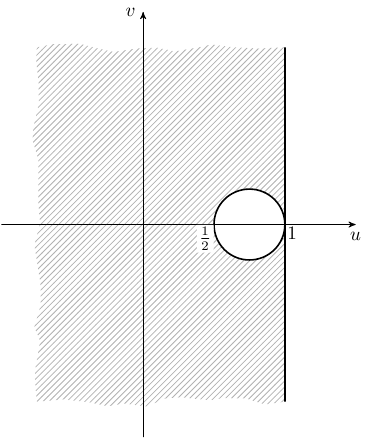# TiKZTikz latex question (Evgeny.Makarov)

#### dwsmith

##### Well-known member
\begin{align}
\frac{1}{4} &< (1 - u)^2 + \left(v + \frac{1}{2}\right)^2\notag\\
\frac{1}{4} &< (1 - u)^2 + \left(v - \frac{1}{2}\right)^2\notag
\end{align}

How can I make this on the complex plane with the appropriate area shaded?

u is the real axis and v the imaginary

#### dwsmith

##### Well-known member
\begin{align}
\frac{1}{4} &< (1 - u)^2 + \left(v + \frac{1}{2}\right)^2\notag\\
\frac{1}{4} &< (1 - u)^2 + \left(v - \frac{1}{2}\right)^2\notag
\end{align}

How can I make this on the complex plane with the appropriate area shaded?

u is the real axis and v the imaginary
I would instead like to graph the image $u < 1$ and $\frac{1}{16} < \left(u - \frac{3}{4}\right)^2 + v^2$

#### Evgeny.Makarov

##### Well-known member
MHB Math Scholar
I would instead like to graph the image $u < 1$ and $\frac{1}{16} < \left(u - \frac{3}{4}\right)^2 + v^2$
This code

Code:
 \usetikzlibrary{arrows,patterns,decorations.pathmorphing}
\begin{tikzpicture}[scale=2.5,>=stealth']
\fill[even odd rule,pattern=north east lines,pattern color=black!30,decoration=random steps]
(1,1.25) -- (1,-1.25) decorate { -- (-.75,-1.25) -- (-.75,1.25) -- cycle}
(.75,0) circle (.25);
\draw[->] (-1,0) -- (1.5,0) node[below] {$u$};
\draw[->] (0,-1.5) -- (0,1.5) node[left] {$v$};
\draw[thick] (.75,0) circle (.25);
\draw[thick] (1,1.25) -- (1,-1.25);
\node[below left,fill=white,inner sep=1pt] at (.5,0) {$\frac{1}{2}$};
\node[below right,inner sep=1pt] at (1,0) {$1$};
\end{tikzpicture}

gives•dwsmith

#### dwsmith

##### Well-known member
I am getting an error on the line \begin{tikzpicture}[scale=2.5,>stealth']

#### Evgeny.Makarov

##### Well-known member
MHB Math Scholar
I am getting an error on the line \begin{tikzpicture}[scale=2.5,>stealth']
First, it should be " >=stealth' ", not " >stealth' ". In fact, options in square brackets are optional here.

Did you say \usepackage{tikz} in the preamble? What is the error message?

•dwsmith

#### dwsmith

##### Well-known member
First, it should be " >=stealth' ", not " >stealth' ". In fact, options in square brackets are optional here.

Did you say \usepackage{tikz} in the preamble? What is the error message?
Ok thanks. Now I have this message 1.114... or =black!30,decoration = random steps]

---------- Post added at 17:38 ---------- Previous post was at 17:36 ----------

Did you say \usepackage{tikz} in the preamble? What is the error message?
Of course

#### Evgeny.Makarov

##### Well-known member
MHB Math Scholar
1.114... or =black!30,decoration = random steps]
This does not look like a complete error message. I am not sure where 1.114 came from.

Try replacing

Code:
\fill[even odd rule,pattern=north east lines,pattern color=black!30,decoration=random steps]
(1,1.25) -- (1,-1.25) decorate { -- (-.75,-1.25) -- (-.75,1.25) -- cycle}
(.75,0) circle (.25);
with

Code:
 \fill[pattern=north east lines]
(1,1.25) -- (1,-1.25) -- (-.75,-1.25) -- (-.75,1.25) -- cycle
(.75,0) circle (.25);
You can also try removing other lines.

Also, I am using PGF/TikZ 2.10. Your version can be generated using the command \pgfversion.

•dwsmith

#### dwsmith

##### Well-known member
This does not look like a complete error message. I am not sure where 1.114 came from.

Try replacing

Code:
\fill[even odd rule,pattern=north east lines,pattern color=black!30,decoration=random steps]
(1,1.25) -- (1,-1.25) decorate { -- (-.75,-1.25) -- (-.75,1.25) -- cycle}
(.75,0) circle (.25);
with

Code:
 \fill[pattern=north east lines]
(1,1.25) -- (1,-1.25) -- (-.75,-1.25) -- (-.75,1.25) -- cycle
(.75,0) circle (.25);
You can also try removing other lines.

Also, I am using PGF/TikZ 2.10. Your version can be generated using the command \pgfversion.

I fixed it typo. How can I make the circle and line at u=1 dashed?

#### Evgeny.Makarov

##### Well-known member
MHB Math Scholar
I fixed it typo.
You copy-pasted the code instead of typing it, right?

How can I make the circle and line at u=1 dashed?
Use [thick,dashed] instead of just [thick] after \draw.

•dwsmith

#### dwsmith

##### Well-known member
I wish I could. At my apt, I can't since my roommate's don't like to pay bills so we have no more internet. I had to read this from my phone.